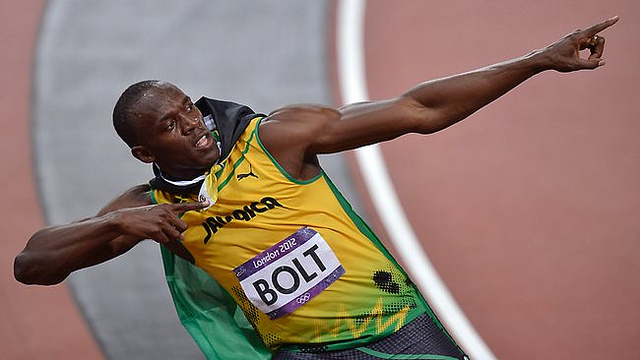# Usain de Bolt-y Wavelength

Usain Bolt, the world champion sprinter, hit a top speed of 27.79 miles per hour at the Olympics. If he has a mass of 94 kg, what was his de Broglie wavelength?

Express your answer as an order of magnitude in units of the Bohr radius $r_{B} = 5.29 \times 10^{-11} \text{m}$. For instance, if your answer was $4 \times 10^{-5} r_{B}$, your should give $-5.$×